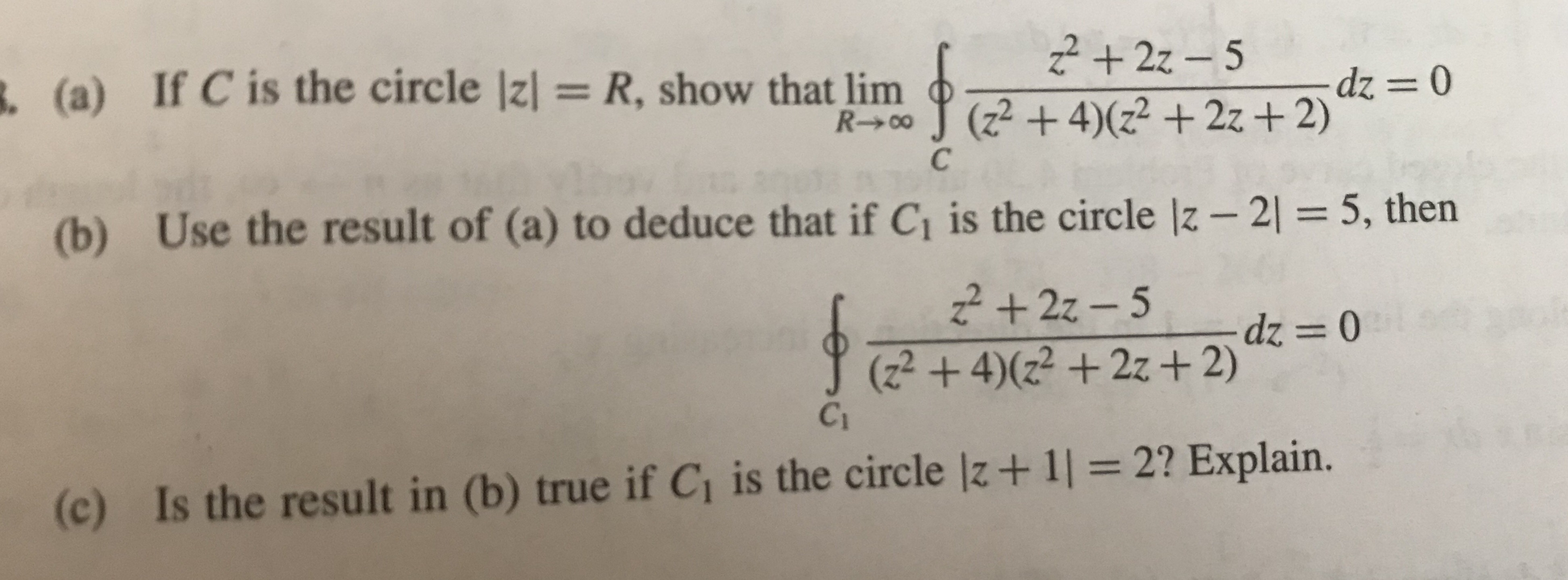# (a)If C is the circle Izl = R, show that linn (2 + 4)(z2 +22+2)(b) Use the result of (a) to deduce that if Ci is the circle lz-25, then(2 +42 +2z+2)Ci(c)Is the result in (b) true if Ci is the circle lz+1l 2? Explain.

Question
33 views

Let z be a complex variable

z=x+iy

question attached in pichelp_outlineImage Transcriptionclose(a) If C is the circle Izl = R, show that linn (2 + 4)(z2 +22+2) (b) Use the result of (a) to deduce that if Ci is the circle lz-2 5, then (2 +42 +2z+2) Ci (c) Is the result in (b) true if Ci is the circle lz+1l 2? Explain. fullscreen
check_circle

Step 1

To examine the complex integral of the given rational function over different contours (circles) in the complex plane

Step 2

First determine all the poles of the integrand;

Step 3

(a) to show that the limit of the integral o...

### Want to see the full answer?

See Solution

#### Want to see this answer and more?

Solutions are written by subject experts who are available 24/7. Questions are typically answered within 1 hour.*

See Solution
*Response times may vary by subject and question.
Tagged in

### Math